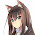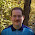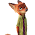## Monday, February 5, 2018

### The wizard monoid

Recent versions of GHC 8.0 provides a `Monoid` instance for `IO` and this post gives a motivating example for why this instance is useful by building combinable "wizard"s.

## Wizards

I'll define a "wizard" as a program that prompts a user "up front" for multiple inputs and then performs several actions after all input has been collected.

Here is an example of a simple wizard:

``````main :: IO ()
main = do
-- First, we request all inputs:
name <- getLine

age <- getLine

-- Then, we perform all actions:
putStrLn ("Your name is: " ++ name)
putStrLn ("Your age is: " ++ age)``````

... which produces the following interaction:

``````What is your name?
Gabriel<Enter>
31<Enter>

... and here is an example of a slightly more complex wizard:

``````import qualified System.Directory

main :: IO ()
main = do
-- First, we request all inputs:
files <- System.Directory.listDirectory "."
putStrLn ("Would you like to delete " ++ file ++ "?")
response <- getLine
case response of
"y" -> return [file]
_   -> return []

let listOfFilesToRemove = concat listOfListOfFilesToRemove

-- Then, we perform all actions:
let removeFile file = do
putStrLn ("Removing " ++ file)
System.Directory.removeFile file
mapM_ removeFile listOfFilesToRemove``````

... which produces the following interaction:

``````Would you like to delete file1.txt?
y<Enter>
Would you like to delete file2.txt?
n<Enter>
Would you like to delete file3.txt?
y<Enter>
Removing file1.txt
Removing file3.txt``````

In each example, we want to avoid performing any irreversible action before the user has completed entering all requested input.

## Modularity

``````main :: IO ()
main = do
-- First, we request all inputs:
name <- getLine

age <- getLine

-- Then, we perform all actions:
putStrLn ("Your name is: " ++ name)
putStrLn ("Your age is: " ++ age)``````

This example is really combining two separate wizards:

• The first wizard requests and displays the user's name
• The second wizard requests and displays the user's age

However, we had to interleave the logic for these two wizards because we needed to request all inputs before performing any action.

What if there were a way to define these two wizards separately and then combine them into a larger wizard? We can do so by taking advantage of the `Monoid` instance for `IO`, like this:

``````import Data.Monoid ((<>))

name :: IO (IO ())
name = do
x <- getLine
return (putStrLn ("Your name is: " ++ x))

age :: IO (IO ())
age = do
x <- getLine
return (putStrLn ("Your age is: " ++ x))

runWizard :: IO (IO a) -> IO a
runWizard request = do
respond <- request
respond

main :: IO ()
main = runWizard (name <> age)``````

This program produces the exact same behavior as before, but now all the logic for dealing with the user's name is totally separate from the logic for dealing with the user's age.

The way this works is that we split each wizard into two parts:

• the "request" (i.e. prompting the user for input)
• the "response" (i.e. performing an action based on that input)

... and we do so at the type-level by giving each wizard the type `IO (IO ())`:

``````name :: IO (IO ())

age :: IO (IO ())``````

The outer `IO` action is the "request". When the request is done the outer `IO` action returns an inner `IO` action which is the "response". For example:

``````--      ↓ The request
name :: IO (IO ())
--          ↑ The response
name = do
x <- getLine
-- ↑ Everything above is part of the outer `IO` action (i.e. the "request")

--      ↓ This return value is the inner `IO` action (i.e. the "response")
return (putStrLn ("Your name is: " ++ x))``````

We combine wizards using the `(<>)` operator, which has the following behavior when specialized to `IO` actions:

``````ioLeft <> ioRight

= do resultLeft  <- ioLeft
resultRight <- ioRight
return (resultLeft <> resultRight)``````

In other words, if you combine two `IO` actions you just run each `IO` action and then combine their results. This in turn implies that if we nest two `IO` actions then we repeat this process twice:

``````requestLeft <> requestRight

= do respondLeft  <- requestLeft
respondRight <- requestRight
return (respondLeft <> respondRight)

= do respondLeft  <- requestLeft
respondRight <- requestRight
return (do
unitLeft  <- respondLeft
unitRight <- respondRight
return (unitLeft <> unitRight) )

-- Both `unitLeft` and `unitRight` are `()` and `() <> () = ()`, so we can
-- simplify this further to:
= do respondLeft  <- requestLeft
respondRight <- requestRight
return (do
respondLeft
respondRight )``````

In other words, when we combine two wizards we combine their requests and then combine their responses.

This works for more than two wizards. For example:

``````request0 <> request1 <> request2 <> request3

= do respond0 <- request0
respond1 <- request1
respond2 <- request2
respond3 <- request3
return (do
respond0
respond1
respond2
respond3 )``````

To show this in action, let's revisit our original example once again:

``````import Data.Monoid ((<>))

name :: IO (IO ())
name = do
x <- getLine
return (putStrLn ("Your name is: " ++ x))

age :: IO (IO ())
age = do
x <- getLine
return (putStrLn ("Your age is: " ++ x))

runWizard :: IO (IO a) -> IO a
runWizard request = do
respond <- request
respond

main :: IO ()
main = runWizard (name <> age)``````

... and this time note that `name` and `age` are awfully similar, so we can factor them out into a shared function:

``````import Data.Monoid ((<>))

prompt :: String -> IO (IO ())
prompt attribute = do
putStrLn ("What is your " ++ attribute ++ "?")
x <- getLine
return (putStrLn ("Your " ++ attribute ++ " is: " ++ x))

runWizard :: IO (IO a) -> IO a
runWizard request = do
respond <- request
respond

main :: IO ()
main = runWizard (prompt "name" <> prompt "age")``````

We were not able to factor out this shared logic back when the logic for the two wizards were manually interleaved. Once we split them into separate logical wizards then we can begin to exploit shared structure to compress our program.

This program compression lets us easily add new wizards:

``````import Data.Monoid ((<>))

prompt :: String -> IO (IO ())
prompt attribute = do
putStrLn ("What is your " ++ attribute ++ "?")
x <- getLine
return (putStrLn ("Your " ++ attribute ++ " is: " ++ x))

runWizard :: IO (IO a) -> IO a
runWizard request = do
respond <- request
respond

main :: IO ()
main = runWizard (prompt "name" <> prompt "age" <> prompt "favorite color")``````

... and take advantage of standard library functions that work on `Monoid`s, like `foldMap` so that we can mass-produce wizards:

``````import Data.Monoid ((<>))

prompt :: String -> IO (IO ())
prompt attribute = do
putStrLn ("What is your " ++ attribute ++ "?")
x <- getLine
return (putStrLn ("Your " ++ attribute ++ " is: " ++ x))

runWizard :: IO (IO a) -> IO a
runWizard request = do
respond <- request
respond

main :: IO ()
main = runWizard (foldMap prompt [ "name", "age", "favorite color", "sign" ])``````

More importantly, we can now easily see at a glance what our program does and ease of reading is a greater virtue than ease of writing.

## Final example

Now let's revisit the file removal example through the same lens:

``````import qualified System.Directory

main :: IO ()
main = do
-- First, we request all inputs:
files <- System.Directory.listDirectory "."
putStrLn ("Would you like to delete " ++ file ++ "?")
response <- getLine
case response of
"y" -> return [file]
_   -> return []

let listOfFilesToRemove = concat listOfListOfFilesToRemove

-- Then, we perform all actions:
let removeFile file = do
putStrLn ("Removing " ++ file)
System.Directory.removeFile file
mapM_ removeFile listOfFilesToRemove``````

We can simplify this using the same pattern:

``````import qualified System.Directory

main :: IO ()
main = do
files <- System.Directory.listDirectory "."
runWizard (foldMap prompt files)

prompt :: FilePath -> IO (IO ())
prompt file = do
putStrLn ("Would you like to delete " ++ file ++ "?")
response <- getLine
case response of
"y" -> return (do
putStrLn ("Removing " ++ file)
System.Directory.removeFile file )
_   -> return (return ())

runWizard :: IO (IO a) -> IO a
runWizard request = do
respond <- request
respond``````

All we have to do is define a wizard for processing a single file, mass-produce the wizard using `foldMap` and the `Monoid` instance for `IO` takes care of bundling all the requests up front and threading the selected files to be removed afterwards.

## Conclusion

This pattern does not subsume all possible wizards that users might want to write. For example, if the wizards depend on one another then this pattern breaks down pretty quickly. However, hopefully this provides an example of you can chain the `Monoid` instance for `IO` with other `Monoid` instance (even itself!) to generate emergent behavior.

1.IIUC, the sentence:
"The first wizard requests and displays the user's age"

should be corrected to:
"The second wizard requests and displays the user's age"

1.Thanks! Fixed

2.A few simplifying substitutions are possible:

runWizard = join
return () = return mempty = mempty
return (return ()) = mempty

3.This is very novel and interesting use of a monoid. What I think would unveil the power of this more is if the result type is more than just a unit.

For example a map/reduce processing type thing, where you read a file in using IO and then the result is a monoid - and the monoid instance is how you want to reduce that thing. For example count the number of "the" in a folder of .txt files.

Then the IO actions could communicate with other 'nodes' to make it distributed, but the monoid that does the counting (... or whatever you want to aggregate) stays the same and doesn't care.

4.Great post! So we've translated it into Japanese impulsively. Sorry for after-the-fact notification. If there's any problem, please notify us:

1.2.3.4.